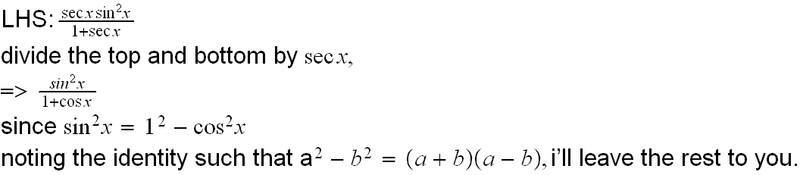# Trig Identity

## Homework Statement

verify the following identity:

Sec(x)Sin2(x)
______________________ = 1 - cos(x)

1 + sec(x)

## Homework Equations

sec(x)=1/cos(x)
sin2(x)=1-cos2(x)

## The Attempt at a Solution

I never know how to start off these problems. I have to take the left side and show that it equals the right by doing trig identities. I try several steps and keep going through an endless loop. I believe I must start of by multiplying 1-sec(x)/1-sec(x)
That way i get

(1-sec(x))(sec(x)sin2(x))
__________________________________

1 - sec2(x)

I'm not sure if i'm starting off correctly

## The Attempt at a Solution

Mark44
Mentor
It would probably be easier to start by replacing the sec(x) terms on the left side with 1/cos(x).

Sec(x)Sin2(x)
______________________ = 1 - cos(x)

1 + sec(x)

ok using the identity sec(x)=1/cos(x)

I get

1/cos(x) * sin2(x)
__________________________

1 + 1/cos(x)

and that =

sin2(x)/cos(x)
______________________

1 + 1/cos(x)

multiply top and bottom by cos(x)

Sin2(x) / 2

Did i do anything wrong?

Thanks

Mark44
Mentor
mcca408 said:
Did i do anything wrong?
Yes. cos(x)*(1 + 1/cos(x)) is not equal to 2. Try again.

I think a trick in handling trigo qns is to convert all to sine and cosine functions.
since sec, csc and even tan functions can be derived from sine and cosine.

Do remember this to help in your future sch works as well.#### Attachments

sin2(x)/cos(x)
______________________

1 + 1/cos(x)

Turn the denominator into $$\frac{cos(x)+1}{cos(x)}$$.

So that would look like $$\frac{\frac{sin^{2}(x)}{cos(x)}}{\frac{cos(x)+1}{cos(x)}}.$$

What icystrike showed is the faster way but since you're already this far, you can try what I suggested.